# Sec 10 Solving Quadratic Equations by Factoring a

• Slides: 10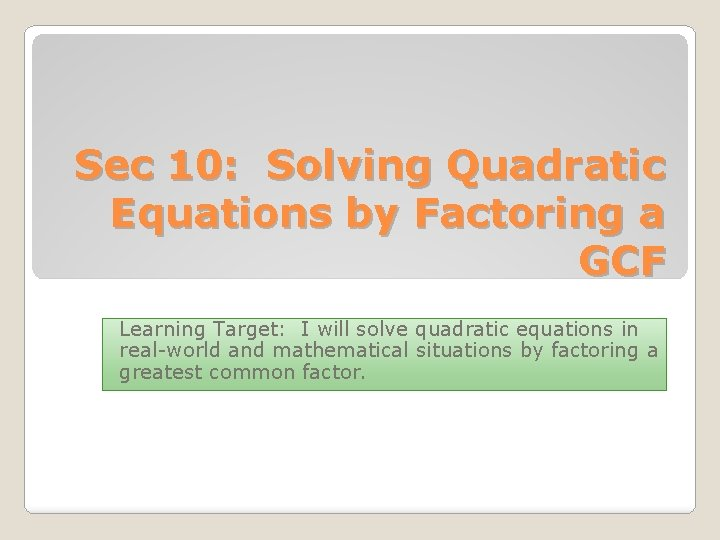Sec 10: Solving Quadratic Equations by Factoring a GCF Learning Target: I will solve quadratic equations in real-world and mathematical situations by factoring a greatest common factor.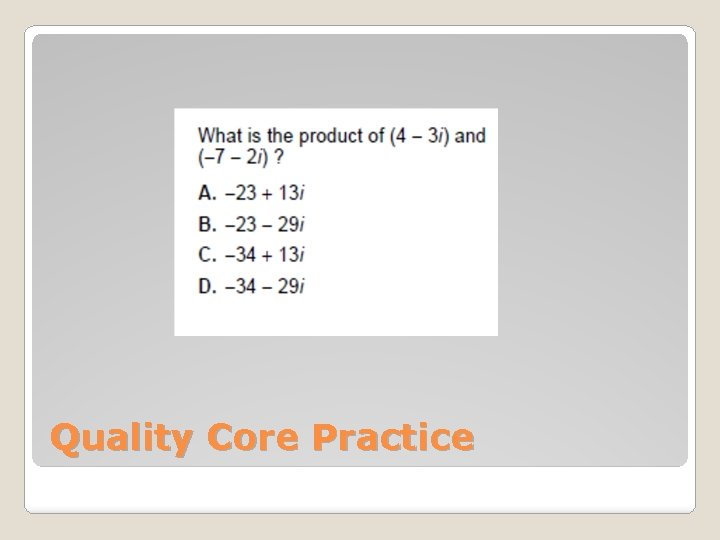Quality Core PracticeTypes of Polynomials Type # of Terms Example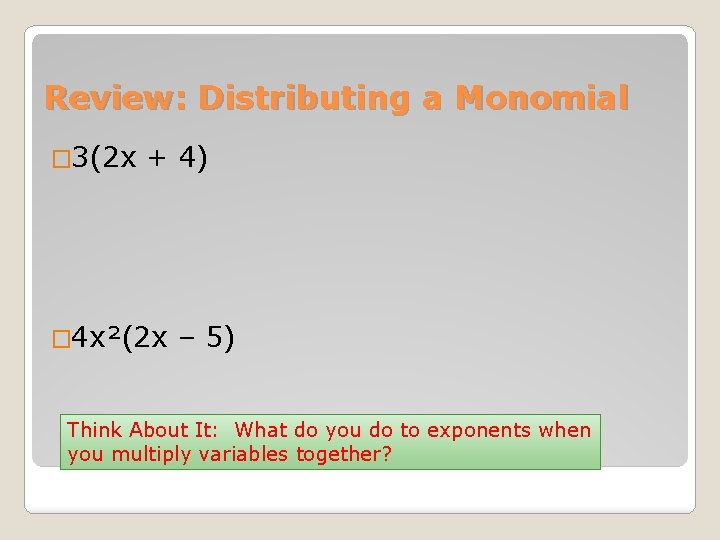Review: Distributing a Monomial � 3(2 x + 4) � 4 x²(2 x – 5) Think About It: What do you do to exponents when you multiply variables together?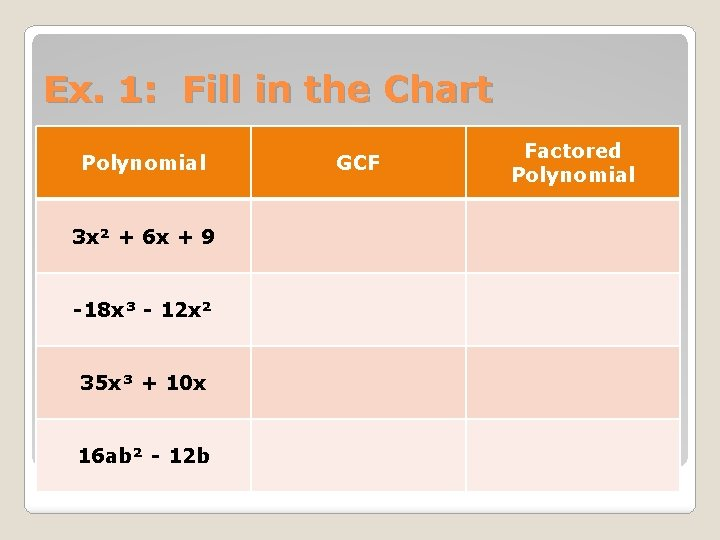Ex. 1: Fill in the Chart Polynomial 3 x² + 6 x + 9 -18 x³ - 12 x² 35 x³ + 10 x 16 ab² - 12 b GCF Factored Polynomial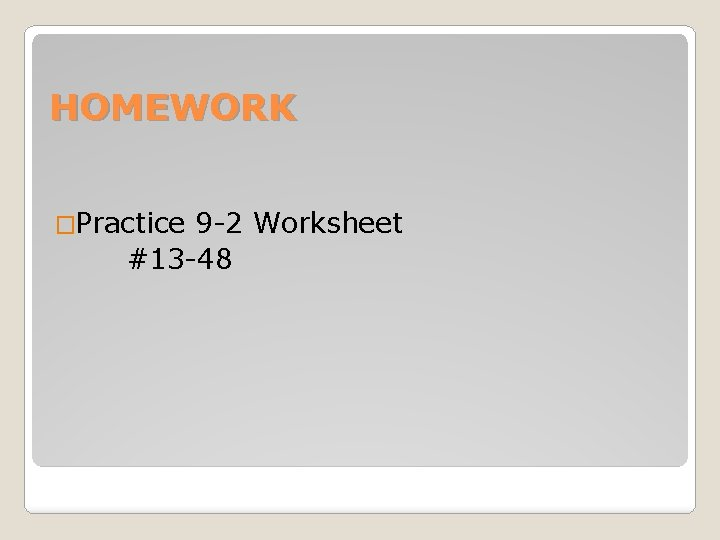HOMEWORK �Practice 9 -2 Worksheet #13 -48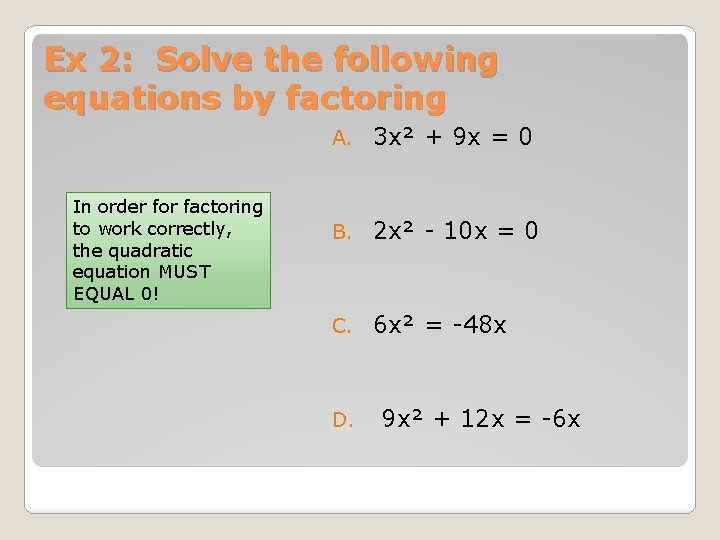Ex 2: Solve the following equations by factoring In order for factoring to work correctly, the quadratic equation MUST EQUAL 0! A. 3 x² + 9 x = 0 B. 2 x² - 10 x = 0 C. 6 x² = -48 x D. 9 x² + 12 x = -6 xEx 3: Ball �Suppose you throw a ball straight up from the ground with a velocity of 80 ft/sec. The height of the ball is given by h = -16 t² + 80 t. A. How many seconds is the ball in the air? B. How high will the ball go?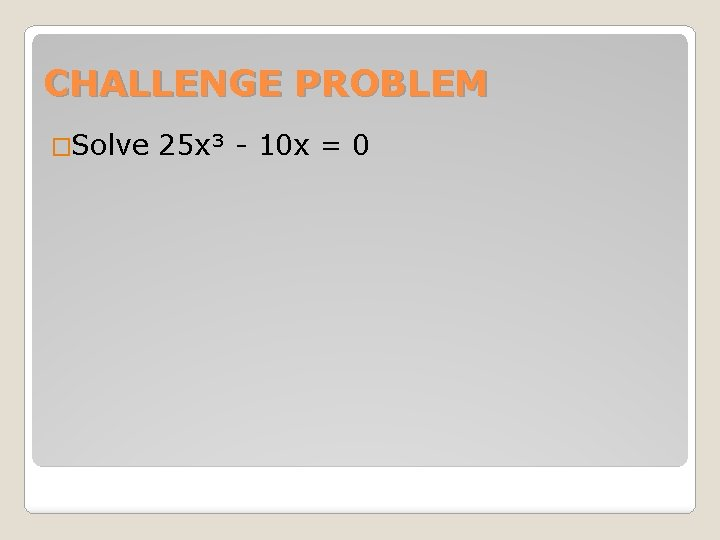CHALLENGE PROBLEM �Solve 25 x³ - 10 x = 0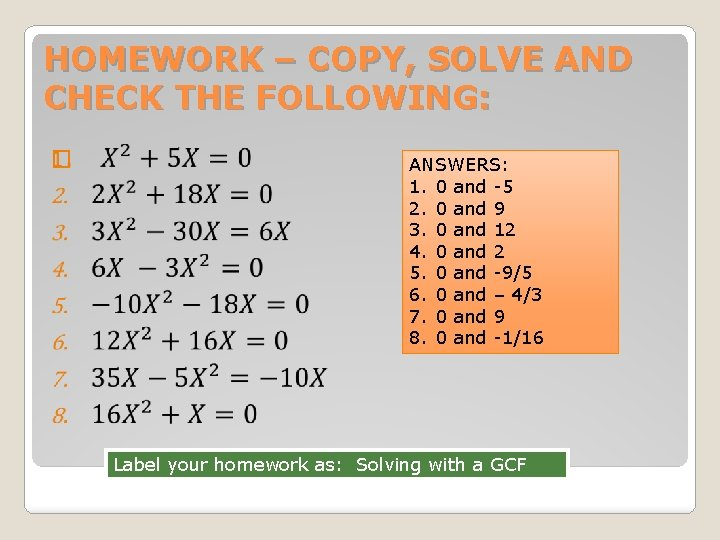HOMEWORK – COPY, SOLVE AND CHECK THE FOLLOWING: � ANSWERS: 1. 0 and -5 2. 0 and 9 3. 0 and 12 4. 0 and 2 5. 0 and -9/5 6. 0 and – 4/3 7. 0 and 9 8. 0 and -1/16 Label your homework as: Solving with a GCF